# 21 Results

View
Selected filters:
• CCSS.Math.Content.HSF-BF.A.1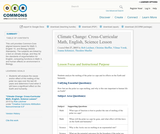Conditions of Use:
Remix and Share
Rating

This unit provides Common-Core aligned lessons based for Math 3, English 10, and Biology (NGSS Standards). The subjects are linked by a text on climate change, and they hit the standards of argumentation for English, comparing functions in Math 3, and human effects on environment in Biology.

Subject:
Applied Science
English Language Arts
Life Science
Mathematics
Material Type:
Activity/Lab
Assessment
Data Set
Diagram/Illustration
Homework/Assignment
Interactive
Lesson Plan
Primary Source
Teaching/Learning Strategy
Author:
Joanna Schimizzi
Christine Sheffler
Rob Leichner
Theodore Mueller
Yilmaz Yoruk
01/28/2016Rating

This lesson unit is intended to help you assess how well students are able to use geometric properties to solve problems. In particular, it will support you in identifying and helping students who have the following difficulties: Solving problems relating to using the measures of the interior angles of polygons; and solving problems relating to using the measures of the exterior angles of polygons.

Subject:
Geometry
Material Type:
Assessment
Lesson Plan
Provider:
Shell Center for Mathematical Education
U.C. Berkeley
Provider Set:
Mathematics Assessment Project (MAP)
04/26/2013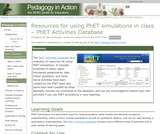Conditions of Use:
Remix and Share
Rating

The PhET Activities Database is a collection of resources for using PhET sims. It includes hundreds of lesson plans, homework assignments, labs, clicker questions, and more. Some activities have been created by the PhET team and some have been created by teachers.

Subject:
Life Science
Physical Science
Material Type:
Activity/Lab
Simulation
Provider:
Science Education Resource Center (SERC) at Carleton College
Provider Set:
Pedagogy in Action
Starting Point (SERC)
Author:
Sam McKagan
08/28/2012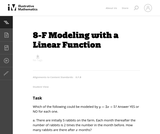Conditions of Use:
No Strings Attached
Rating

The primary purpose of this task is to elicit common misconceptions that arise when students try to model situations with linear functions. This task, being multiple choice, could also serve as a quick assessment to gauge a class' understanding of modeling with linear functions.

Subject:
Mathematics
Functions
Material Type:
Activity/Lab
Provider:
Illustrative Mathematics
Provider Set:
Illustrative Mathematics
Author:
Illustrative Mathematics
05/01/2012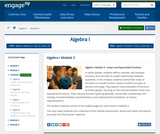Conditions of Use:
Remix and Share
Rating

In earlier grades, students define, evaluate, and compare functions and use them to model relationships between quantities. In this module, students extend their study of functions to include function notation and the concepts of domain and range. They explore many examples of functions and their graphs, focusing on the contrast between linear and exponential functions. They interpret functions given graphically, numerically, symbolically, and verbally; translate between representations; and understand the limitations of various representations.

Subject:
Algebra
Material Type:
Module
Provider:
New York State Education Department
Provider Set:
EngageNY
09/17/2013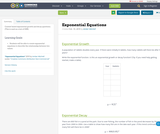Conditions of Use:
Remix and Share
Rating

Context based exponential growth and decay questions.

(This is used as a test of OER)

Subject:
Mathematics
Material Type:
Homework/Assignment
Author:
Amber Mitchell
02/19/2019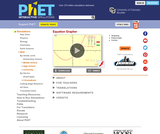Conditions of Use:
No Strings Attached
Rating

Learn about graphing polynomials. The shape of the curve changes as the constants are adjusted. View the curves for the individual terms (e.g. y=bx ) to see how they add to generate the polynomial curve.

Subject:
Algebra
Material Type:
Simulation
Provider:
Provider Set:
PhET Interactive Simulations
Author:
Michael Dubson
Mindy Gratny
Trish Loeblein
11/16/2007Conditions of Use:
Remix and Share
Rating

This resource was created by the Washington Office of Superintendent of Public Instruction.

Subject:
Mathematics
Material Type:
Assessment
Homework/Assignment
Lesson
Author:
Hannah Hynes-Petty
03/31/2020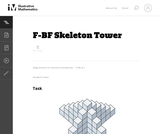Conditions of Use:
No Strings Attached
Rating

This problem is a quadratic function example. The other tasks in this set illustrate F.BF.1a in the context of linear (Kimi and Jordan), exponential (Rumors), and rational (Summer Intern) functions.

Subject:
Mathematics
Functions
Material Type:
Activity/Lab
Provider:
Illustrative Mathematics
Provider Set:
Illustrative Mathematics
Author:
Illustrative Mathematics
05/01/2012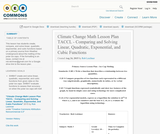Conditions of Use:
Remix and Share
Rating

This lesson has students create, compare, and solve linear, quadratic, exponential, and cubic functions based on a primary source from Weather Underground about the melting of the polar ice caps. If the formatting is an issue, contact me at rob.leichner@gmail.com for a Google drive link to the lesson plan.

Subject:
English Language Arts
Mathematics
Material Type:
Activity/Lab
Assessment
Data Set
Homework/Assignment
Interactive
Lesson Plan
Primary Source
Author:
Rob Leichner
01/28/2016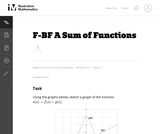Conditions of Use:
No Strings Attached
Rating

The intent of this problem is to have students think about how function addition works on a fundamental level, so formulas have been omitted on purpose.

Subject:
Mathematics
Functions
Material Type:
Activity/Lab
Provider:
Illustrative Mathematics
Provider Set:
Illustrative Mathematics
Author:
Illustrative Mathematics
05/01/2012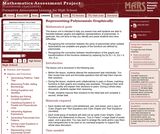Rating

This lesson unit is intended to help teachers assess how well students are able to translate between graphs and algebraic representations of polynomials. In particular, this unit aims to help you identify and assist students who have difficulties in: recognizing the connection between the zeros of polynomials when suitable factorizations are available, and graphs of the functions defined by polynomials; and recognizing the connection between transformations of the graphs and transformations of the functions obtained by replacing f(x) by f(x + k), f(x) + k, -f(x), f(-x).

Subject:
Mathematics
Material Type:
Assessment
Lesson Plan
Provider:
Shell Center for Mathematical Education
U.C. Berkeley
Provider Set:
Mathematics Assessment Project (MAP)
04/26/2013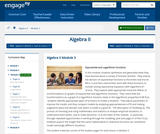Conditions of Use:
Remix and Share
Rating

In this module, students synthesize and generalize what they have learned about a variety of function families.  They extend the domain of exponential functions to the entire real line (N-RN.A.1) and then extend their work with these functions to include solving exponential equations with logarithms (F-LE.A.4).  They explore (with appropriate tools) the effects of transformations on graphs of exponential and logarithmic functions.  They notice that the transformations on a graph of a logarithmic function relate to the logarithmic properties (F-BF.B.3).  Students identify appropriate types of functions to model a situation.  They adjust parameters to improve the model, and they compare models by analyzing appropriateness of fit and making judgments about the domain over which a model is a good fit.  The description of modeling as, the process of choosing and using mathematics and statistics to analyze empirical situations, to understand them better, and to make decisions, is at the heart of this module.  In particular, through repeated opportunities in working through the modeling cycle (see page 61 of the CCLS), students acquire the insight that the same mathematical or statistical structure can sometimes model seemingly different situations.

Subject:
Algebra
Material Type:
Module
Provider:
New York State Education Department
Provider Set:
EngageNY
09/16/2014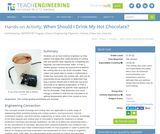Conditions of Use:
Rating

Students act as food science engineers as they explore and apply their understanding of cooling rate and specific heat capacity by completing two separate, but interconnected, tasks. In Part 1, student groups conduct an experiment to explore the cooling rate of a cup of hot chocolate. They collect and graph data to create a mathematical model that represents the cooling rate, and use an exponential decay regression to determine how long a person should wait to drink the cup of hot chocolate at an optimal temperature. In Part 2, students investigate the specific heat capacity of the hot chocolate. They determine how much energy is needed to heat the hot chocolate to an optimal temperature after it has cooled to room temperature. Two activity-guiding worksheets are included.

Subject:
Mathematics
Algebra
Statistics and Probability
Physical Science
Chemistry
Material Type:
Activity/Lab
Provider:
TeachEngineering
Provider Set:
TeachEngineering
Author:
Brian Palacios
02/17/2017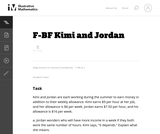Conditions of Use:
No Strings Attached
Rating

In the middle grades, students have lots of experience analyzing and comparing linear functions using graphs, tables, symbolic expressions, and verbal descriptions. In this task, students may choose a representation that suits them and then reason from within that representation.

Subject:
Mathematics
Functions
Material Type:
Activity/Lab
Provider:
Illustrative Mathematics
Provider Set:
Illustrative Mathematics
Author:
Illustrative Mathematics
05/01/2012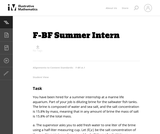Conditions of Use:
No Strings Attached
Rating

In this task students must figure out how much fresh water you must add to get a particular salt concentration in aquarium water.

Subject:
Mathematics
Functions
Material Type:
Activity/Lab
Provider:
Illustrative Mathematics
Provider Set:
Illustrative Mathematics
Author:
Illustrative Mathematics
05/01/2012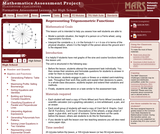Rating

This lesson unit is intended to help teachers assess how well students are able to: model a periodic situation, the height of a person on a Ferris wheel, using trigonometric functions; and interpret the constants a, b, c in the formula h = a + b cos ct in terms of the physical situation, where h is the height of the person above the ground and t is the elapsed time.

Subject:
Functions
Material Type:
Assessment
Lesson Plan
Provider:
Shell Center for Mathematical Education
U.C. Berkeley
Provider Set:
Mathematics Assessment Project (MAP)
04/26/2013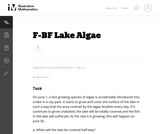Conditions of Use:
No Strings Attached
Rating

The purpose of this task is to introduce students to exponential growth. While the context presents a classic example of exponential growth, it approaches it from a non-standard point of view. Instead of giving a starting value and asking for subsequent values, it gives an end value and asks about what happened in the past. The simple first question can generate a surprisingly lively discussion as students often think that the algae will grow linearly.

Subject:
Mathematics
Functions
Material Type:
Activity/Lab
Provider:
Illustrative Mathematics
Provider Set:
Illustrative Mathematics
Author:
Illustrative Mathematics
05/01/2012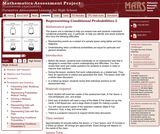Rating

This lesson unit is intended to help teachers assess how well students understand conditional probability, and, in particular, to help teachers identify and assist students who have the following difficulties: representing events as a subset of a sample space using tables and tree diagrams; and understanding when conditional probabilities are equal for particular and general situations.

Subject:
Statistics and Probability
Material Type:
Assessment
Lesson Plan
Provider:
Shell Center for Mathematical Education
U.C. Berkeley
Provider Set:
Mathematics Assessment Project (MAP)
04/26/2013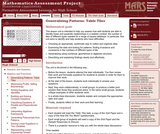Rating

This lesson unit is intended to help teachers assess how well students are able to identify linear and quadratic relationships in a realistic context: the number of tiles of different types that are needed for a range of square tabletops. In particular, this unit aims to identify and help students who have difficulties with: choosing an appropriate, systematic way to collect and organize data; examining the data and looking for patterns; finding invariance and covariance in the numbers of different types of tile; generalizing using numerical, geometrical or algebraic structure; and describing and explaining findings clearly and effectively.

Subject:
Algebra
Geometry
Measurement and Data
Material Type:
Assessment
Lesson Plan
Provider:
Shell Center for Mathematical Education
U.C. Berkeley
Provider Set:
Mathematics Assessment Project (MAP)